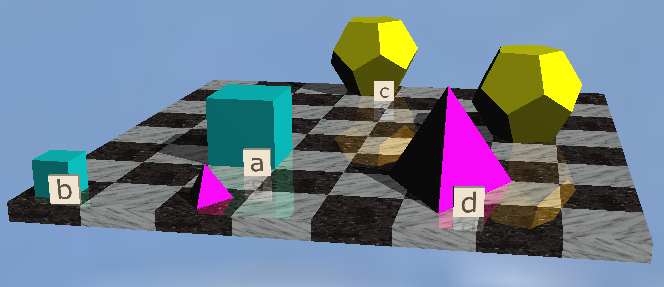# LP&L Trees 4 Propositional Samples

Logical System
###### 1/28/2020

John Barwise and John Etchemendy,  Language, Proof and Logic

For the Tarski's World part of LP&L, a standard interpretation is used of various sized polyhedra on a chessboard, for example,Here we are just trying a few samples to see if the software is running correctly.

## Exercise

[In the widgets below:- Click once to select, click again to un-select. Select one item to extend the Tree, select two to close a branch. Select one or two for extending by Analytic Consequence (depending what inference you are trying for). Select two for extending with identity.]

1. RightOf(b,c), LeftOf(d,e), b=d ∴ LeftOf(c,e) (p.52)

2. SameRow(b,c), SameRow(a,d), SameRow(d,f), FrontOf(a,b) ∴ FrontOf(f,c) (p.66)

3. (Tet(a)∧Small(a)) ∨ Small(b) ∴ Small(a)∨ Small(b)  (p.113)

4. Cube(a)∨ Cube(b), Dodec(c)∨ Dodec(d), ¬Cube(a)∨ ¬Dodec(c) ∴ Cube(b)∨ Dodec(d) (p.117)

5. LeftOf(a,b)∨RightOf(a,b), BackOf(a,b)∨¬LeftOf(a,b), FrontOf(b,a)∨ ¬RightOf(a,b), SameCol(c,a)∧SameRow(c,b) ∴ BackOf(a,b)  (p.135)

6. ¬Cube(b)→Small(b), Small(c)→(Small(d)∨Small(e)), Small(d)→¬Small(c), Cube(b)→¬Small(e) ∴ Small(c)→Small(b)   (p.213)